# Test Prep Plan - Take a practice test

Take this practice test to check your existing knowledge of the course material. We'll review your answers and create a Test Prep Plan for you based on your results.
How Test Prep Plans work
1
2Based on your results, we'll create a customized Test Prep Plan just for you!
3Study smarter
Study more effectively: skip concepts you already know and focus on what you still need to learn.

# Properties of Derivatives Chapter Exam

Exam Instructions:

Choose your answers to the questions and click 'Next' to see the next set of questions. You can skip questions if you would like and come back to them later with the yellow "Go To First Skipped Question" button. When you have completed the practice exam, a green submit button will appear. Click it to see your results. Good luck!

### Page 1

#### Question 1 1. Find the derivative of f(x).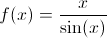#### Question 2 2. Which is greater, the derivative at a or the derivative at b?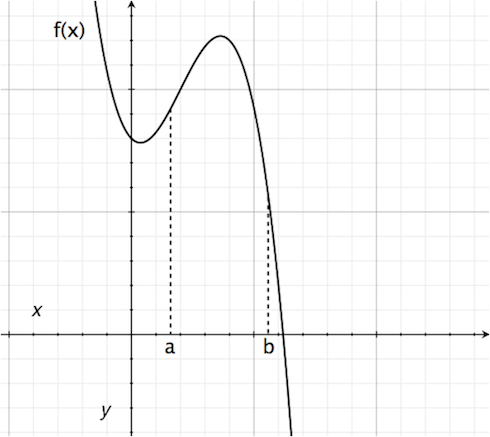#### Question 3 3. At how many points on the graph below is the derivative of f(x)=0?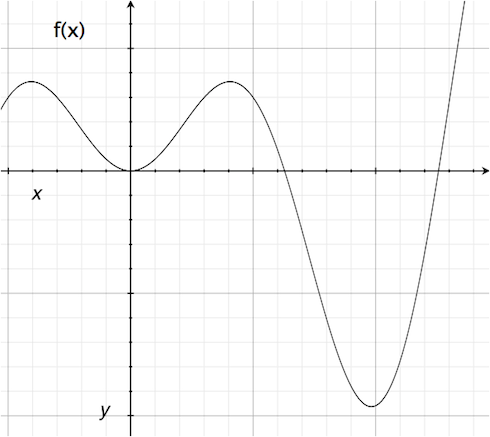#### Question 4 4. Where is f(x) differentiable?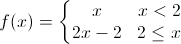#### Question 5 5. Find y'.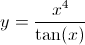### Page 2

#### Question 6 6. The derivative of f(x) is equal to 0 at how many points on the graph below?#### Question 7 7. Calculate the derivative of f(x), and evaluate it at x=2.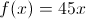#### Question 8 8. Where is f(x) differentiable?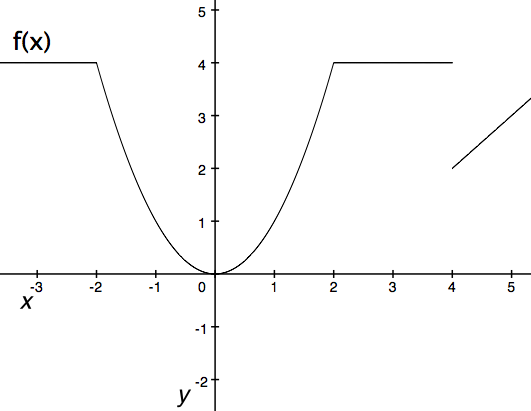#### Question 9 9. What is the derivative of f(z)?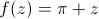#### Question 10 10. Where t= time, what is the derivative of f(t)?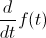### Page 3

#### Question 11 11. Consider the graph and question below..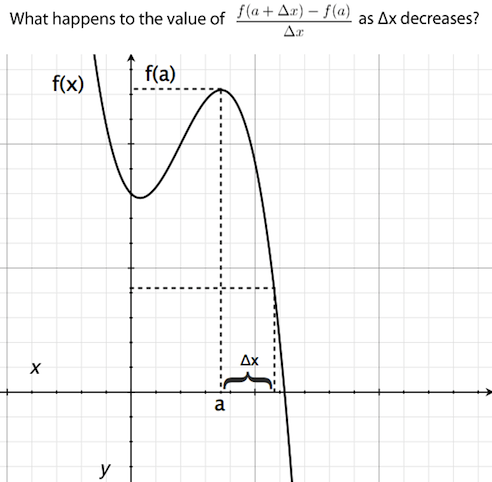#### Question 12 12. Which of the following statements is true about the derivative of f(x)?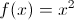#### Question 14 14. What is the derivative of y?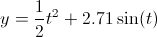### Page 4

#### Question 16 16. Find f'(t) given the function f(t):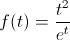#### Question 17 17. Where is f(x) differentiable?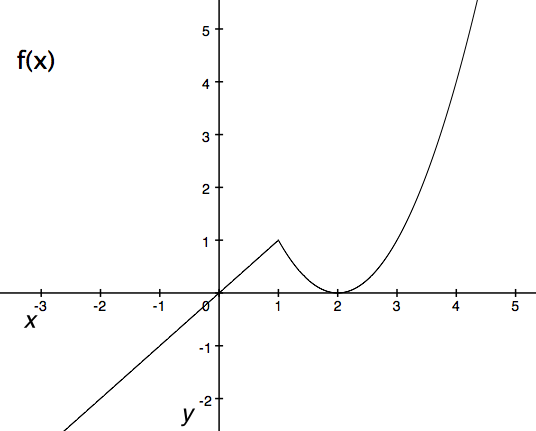#### Question 18 18. Evaluate the following: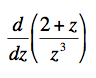#### Question 19 19. Where is y differentiable?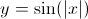#### Question 20 20. What is the derivative of f(x)?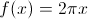### Page 5

#### Question 21 21. What is the derivative of f(x)?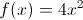#### Question 22 22. What is the value of f(x) at the red circle?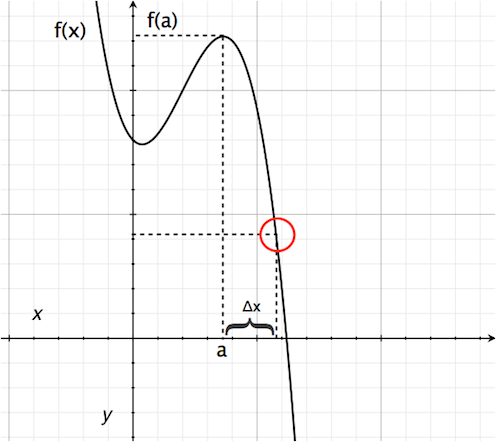#### Question 23 23. Which of the following statements is true, given g(t) below?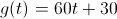#### Question 24 24. What is the derivative of g(t)? Assume C is a constant.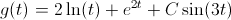#### Question 25 25. The derivative below may have been calculated from which function f(x)?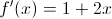### Page 6

#### Question 26 26. Calculate the derivative of g(t).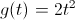#### Question 28 28. Where is f(x) differentiable?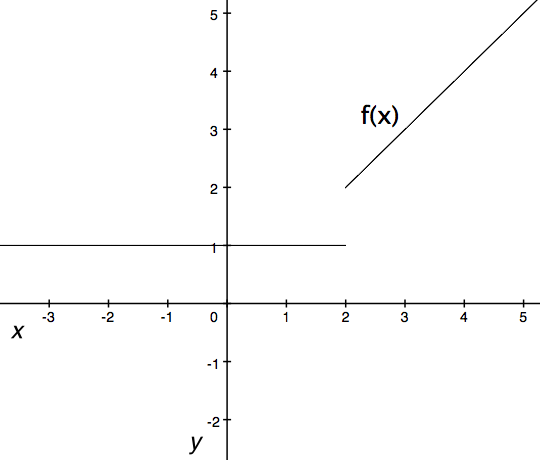#### Question 29 29. What is the derivative of the following?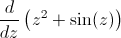#### Question 30 30. Differentiate the following function: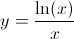#### Properties of Derivatives Chapter Exam Instructions

Choose your answers to the questions and click 'Next' to see the next set of questions. You can skip questions if you would like and come back to them later with the yellow "Go To First Skipped Question" button. When you have completed the practice exam, a green submit button will appear. Click it to see your results. Good luck!

Support